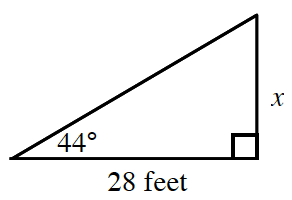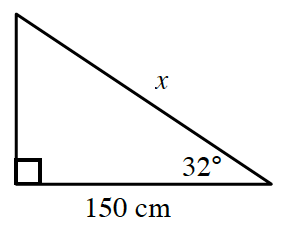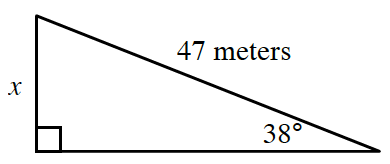### Home > CCA2 > Chapter Ch7 > Lesson 7.1.1 > Problem7-5

7-5.

In each of the following triangles, find the length of the side labeled $x$.

 a.b.c.Hint (a):Which trigonometric relationship uses the opposite and adjacent sides?More Help (a):$\tan (44^\circ)=\frac{x}{28}$Answer (a):$27.04\ \text{feet}$ Hint (b):Identify the sides involved and choose the appropriate trigonometric relationship. Hint (c):See part (b).